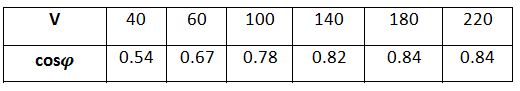# Power factor in different voltages

Hi all.

In the following circuit, z is an inductive load (I mean inductance + resistance).Here is the table of cosφ measured by power factor meter in different voltages:Why it's increasing? Isn't cosφ independent of the voltage of the autotransformer?

The inductance of the AutoTrans is changing as you adjust the voltage - try the same test with purely resistive load.

Isn't power factor meter just measuring load voltage? If yes, how can inductance of the AutoTrans effect?
(For purely resistive load, the increase was lesser.)

The PF meter is really measuring the Phase angle between the V and I - this relationship is affected by everything in the circuit - including the AutoTrans.

So if your load was a pure resistor - the phase shift would be due to the Inductance of the Autotrans - at the different adjustment points. Techincally the total impedance of the source feeding the AutoTrans will have an effect as well - but not as pronounced as the data you are showing, unless you have a "weak" source like a isolation transformer - etc.

What do you mean by V?
How the inductance of AutoTrans changes, increase or decrease?
Thanks again

V voltage and I current - the basic PF meter really measures the phase angle between the two - not a real power factor - but close enough for a lab exercise.

As for will the Inductance increase or decrease - I do not know - but looking at your data - what is your guess?